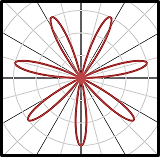# Sketch the region and use integration to find its area. The region bounded by all leaves of the...

## Question:

Sketch the region and use integration to find its area.

The region bounded by all leaves of the rose {eq}\,r = 3\sin 7\theta {/eq}

## Area by a Polar Function:

The area bounded by a certain polar function can be determined using the integration method and with the formula given as {eq}A = \dfrac{1}{2} \int [f(\theta)]^2 d\theta {/eq}. Note that the limits must be defined correctly in order to solve for the desired enclosed area.

Become a Study.com member to unlock this answer!

The given polar function has the figure:We calculate region bounded by all leaves using the equation

{eq}A = \dfrac{1}{2} \int [f(\theta)]^2...Evaluating Definite Integrals Using the Fundamental Theorem

from

Chapter 16 / Lesson 2
1.2K

In calculus, the fundamental theorem is an essential tool that helps explain the relationship between integration and differentiation. Learn about evaluating definite integrals using the fundamental theorem, and work examples to gain understanding.